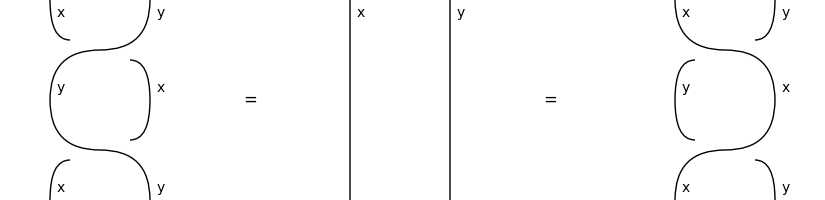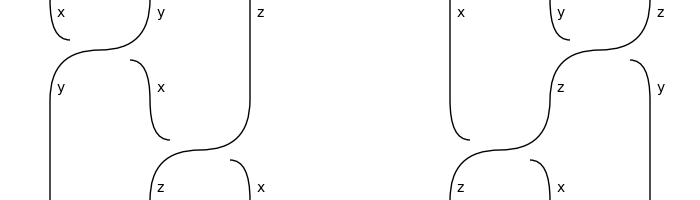# braided#

The free braided category, i.e. diagrams with braids.

## Summary#

 `Diagram` A braided diagram is a monoidal diagram with `Braid` boxes. `Box` A braided box is a monoidal box in a braided diagram. `Braid` The braiding of atomic types `left` and `right`. `Sum` A braided sum is a monoidal sum and a braided box. `Category` A braided category is a monoidal category with a method `braid`. `Functor` A braided functor is a monoidal functor that preserves braids.

Functions

 `hexagon` Take a `factory` for braids of atomic types and extend it recursively.

## Axioms#

Braids have their dagger as inverse, up to `Diagram.simplify()`.

```>>> x, y, z = map(Ty, "xyz")
>>> LHS = Braid(x, y) >> Braid(x, y)[::-1]
>>> RHS = Braid(y, x)[::-1] >> Braid(y, x)
>>> assert LHS.simplify() == Id(x @ y) == RHS.simplify()
```
```>>> from discopy.drawing import Equation
>>> Equation(LHS, Id(x @ y), RHS).draw(
...     path='docs/_static/braided/inverse.png', figsize=(5, 2))
```The hexagon equations hold on the nose.

```>>> left_hexagon = Braid(x, y) @ z >> y @ Braid(x, z)
>>> assert left_hexagon == Diagram.braid(x, y @ z)
>>> right_hexagon = x @ Braid(y, z) >> Braid(x, z) @ y
>>> assert right_hexagon == Diagram.braid(x @ y, z)
```
```>>> Equation(left_hexagon, right_hexagon, symbol='').draw(
...     space=2, path='docs/_static/braided/hexagons.png', figsize=(5, 2))
```